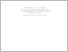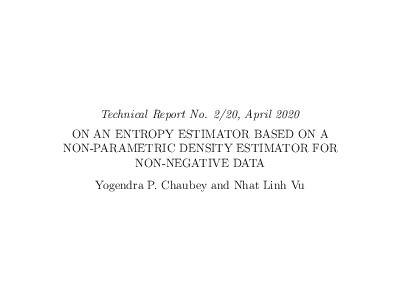Title:

# On An Entropy Estimator Based On a Non-parametric Density Estimator For Non-negative Data

Chaubey, Yogendra and Vu, Nhat Linh (2020) On An Entropy Estimator Based On a Non-parametric Density Estimator For Non-negative Data. Technical Report. UNSPECIFIED. (Unpublished)Preview
Text (application/pdf)
2020_2_Yogen_TechReport.pdf - Draft Version
673kB

## Abstract

In the recent decades, entropy has become more and more essential in statistics and machine learning. It features in many applications involving data transmission, cryptography, signal processing, network theory, bio-informatics, and so on. A large number of estimators for entropy have been proposed in the past ten years. Here we focus on entropy estimation for non-negative random variables. Specifically, the use of entropy estimator based on Poisson-weights density estimator is found to be of interest. We establish some asymptotic properties of the resulting estimators and present a simulation study comparing these with well known estimators in literature.

Divisions: Concordia University > Faculty of Arts and Science > Mathematics and Statistics Monograph (Technical Report) No Chaubey, Yogendra and Vu, Nhat Linh 28 April 2020 Natural Sciences and Engineering Research Council information theory, entropy estimator, non-parametric density estimator, asymptotic properties. 986758 YOGENDRA CHAUBEY 07 May 2020 13:59 07 May 2020 13:59

## References:

References
 Ahmad, I.A. and Lin, P.E. (1989). A nonparametric estimation of the entropy for
absolutely continuous distributions. IEEE Trans. Inform. Theory, 36, 688-692.
 Bagai, I. and Prakasa Rao, B.L.S. (1996). Kernel type density estimates for positive valued random variables. Sankhya Ser. A, 57, 56-67.
 Beirlant, J., Dudewicz, E.J., Gyorfi, L.,van de Meulen, E.C. (1997). Nonparametric entropy estimation. An overview. International Journal of Mathematical and Statistical Sciences, 6, 17-39.
 Bouezmarni, T. and Scaillet, O. (2005). Consistency of asymmetric kernel density estimators and smoothed histograms with application to income data. Econometric Theory, 21, 390-412.
 Bouzebda, S., Elhattab, I., Keziou, A., and Lounis, T. (2013). New entropy estimator with an application to test of normality. Communication in Statistics-Theory and Methods, 42, 2245-2270.
 Chaubey, Y.P., Li, J., Sen, A. and Sen, P.K. (2012). A new smooth density estimator for non-negative random variables. Journal of the Indian Statistical Association, 50, 83-104.
 Chaubey, Y.P. and Mudholkar, G.S. (2013). A rationale for maximizing likelihood and related alternatives to maximum likelihood estimator. Investigations in Mathematical Sciences, 3, 1-15.
 Chaubey, Y.P. and Sen, P.K. (1996). On smooth estimation of survival and density functions. Statistics and Decisions, 14, 1{22.
 Chaubey, Y.P. and Sen, P.K. (2009). On the selection of the smoothing parameter in Poisson smoothing of histogram estimator: Computational aspects. Pakistan Journal of Statistics, 25, 385-401.
 Chaubey, Y.P., Sen, P.K. and Li, J. (2010). Smooth density estimation for length-biased data. Journal of The Indian Society of Agricultural Statistics, 64, 145-155.
 Chen, S.X. (2000). Probability Density Function Estimation Using Gamma Kernels. Annals of the Institute of Statistical Mathematics, 52, 471-480.
 Cheng, C. and Parzen, E. (1997). Unibiased estimators of smooth quantile and quantile density funnctions. Journal of Statistical Planning and Inference, 59, 291-307.
 Cover, T.M. and Thomas, J.A. (1991). Elements of Information Theory, Wiley, New York.
 Eggermont, P.P.B. and LaRiccia, V.N. (1999). Best asymptotic normality of the kernel density entropy estimator for smooth densities. IEEE Transactions On Information Theory, 45, 1321-1324.
 Gyorfi, L. and van der Meulen, E.C. (1987). Density-free convergence properties of various estimators of the entropy. Comput. Statist. Data Anal., 5, 425-436.
 Gyorfi, L. and van der Meulen, E.C. (1990). An entropy estimate based on a kernel density estimation, Colloq. Math. Soc. J. Bolyai no. 57. In Limit Theorems in Probability and Statistics, I. Berkes, E. Csaki and P. Revesz, Eds., North-Holland: Amsterdam, The Netherlands.
 Hall, P. and Morton, S.C. (1993). On the estimation of entropy. Ann. Inst. Statist. Math., 45, 69-88.
 Jaynes, E.T. (1957a). Information theory and statistical mechanics-I. The Physical Review, 106, 620-630.
 Jaynes, E.T. (1957b). Information theory and statistical mechanics-II. The Physical Review, 108, 171-190.
 Karunamuni, R.J. and Alberts, T. (2005). On boundary correction in kernel density estimation. Statistical Methodology, 2, 191-212.
 Scaillet, O. (2004). Density estimation using inverse and reciprocal inverse Gaussian kernels. Nonparametric Statistics, 16, 217-226.
 Shannon, C.E. (1948). A mathematical theory of communication. Bell System Tech. J., 27, 379-423. Reprinted in C. E . Shannon and W. Weaver, The Mathematical Theory of Communication, University of Illinois Press, Urbana.
 Shwartz, S., Zibulevsky, M. and Schechner, Y.Y. (2005). Fast kernel entropy estimation and optimization. Signal Processing, 85, 1045-1058.
 Van Es, B. (1992). Estimating functionals related to a density by a class of statistics based on spacings. Scandinavian Journal of Statistics, 19, 61-72.
 Vasicek, O. (1976). A test for normality based on sample entropy. Journal of the Royal Statistical Society, 38, 54-59.
All items in Spectrum are protected by copyright, with all rights reserved. The use of items is governed by Spectrum's terms of access.

Repository Staff Only: item control pageResearch related to the current document (at the CORE website)
Back to top